# QtiPlot - Data Analysis and Scientific Visualisation

## Analytical Surfaces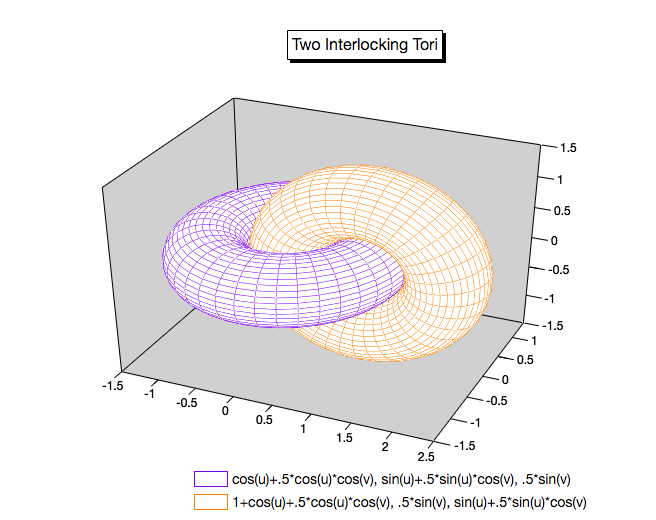QtiPlot can display multiple surfaces in the same 3D plot window. This example shows two interlocking tori. Their parametric equations are given bellow, with u and v being defined in the interval [-π, π]. Download project file >>

`X1(u,v)=cos(u)+.5*cos(u)*cos(v)`
`Y1(u,v)=sin(u)+.5*sin(u)*cos(v)`
`Z1(u,v)=.5*sin(v)`
`X2(u,v)=1+cos(u)+.5*cos(u)*cos(v)`
`Y2(u,v)=.5*sin(v)`
`Z2(u,v)=sin(u)+.5*sin(u)*cos(v)`The 3D parametric surface in this example is a seashell. It can be easily created in QtiPlot using the parametric equations bellow:

`X(u,v)=(1-v/(2*pi))*sin(3*v)*(1+cos(u))+0.5*sin(3*v)`

`Y(u,v)=(1-v/(2*pi))*cos(3*v)*(1+cos(u))+0.5*cos(3*v)`

` Z(u,v)=6*v/(2*pi)+(1-v/(2*pi))*sin(u)`

where u and v are defined in the interval [0, 2π]. Download project file >>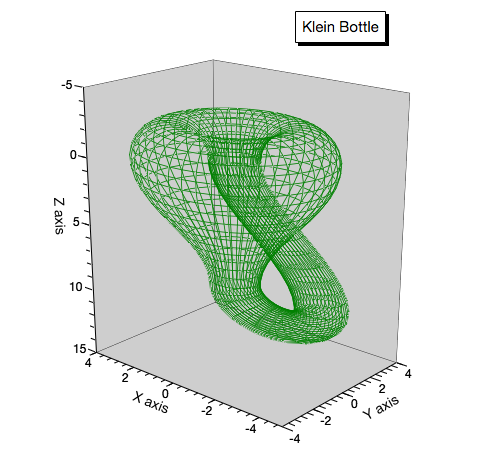QtiPlot can display very complicated 3D surfaces, like the Klein bottle. Its parametric equations are given bellow, with u being defined in the interval [0, 2π] and v in the interval [0, 4π]. Download project file >>

`X(u,v)=v<2*pi?(2.5-1.5*cos(v))*cos(u):(v<3*pi?-2+(2+cos(u))*cos(v):-2+2*cos(v)-cos(u))`

`Y(u,v)=v<2*pi?(2.5-1.5*cos(v))*sin(u):sin(u)`

`Z(u,v)=v<pi?-2.5*sin(v):(v<2*pi?3*v-3*pi:(v<3*pi?(2+cos(u))*sin(v)+3*pi:-3*v+12*pi))`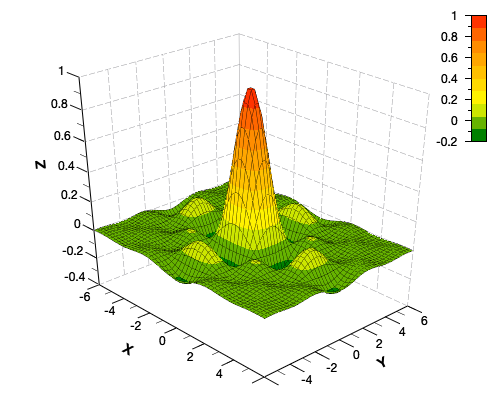This example shows a user defined surface created by QtiPlot from the analytical function bellow:

`F(x,y)=sin(x)*cos(x)*sin(y)*cos(y)/(x*y)`

## Wireframe Plots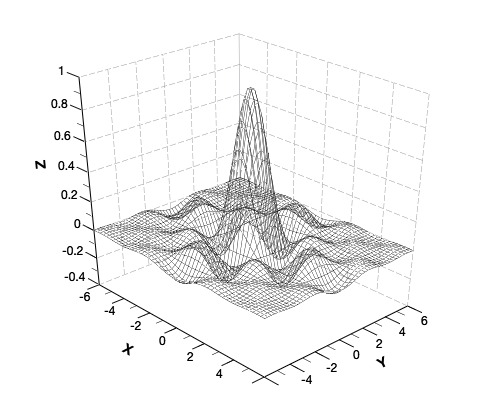Wireframe plots are relatively simple and fast to render and can be used in cases where a high screen frame rate is needed (for instance, when working with a particularly complex 3D data model).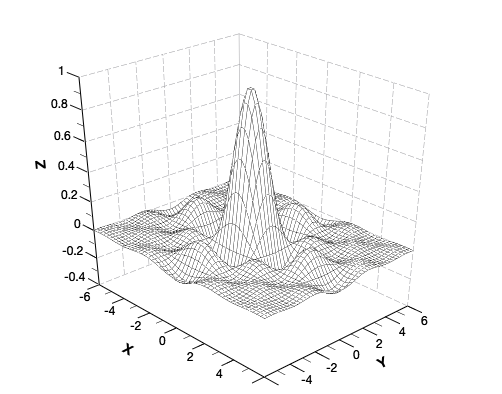QtiPlot can perform hidden-line removal on wireframe plots for a more realistic visualisation of 3D data.

## 3D Bars & Surfaces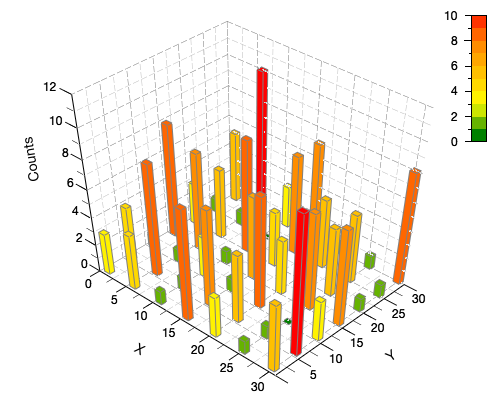A 3D bar chart is a type of graph that presents categorical data with rectangular bars with heights or lengths proportional to the values that they represent.

This example displays the histogram of some bidimensional data as a 3D bar chart.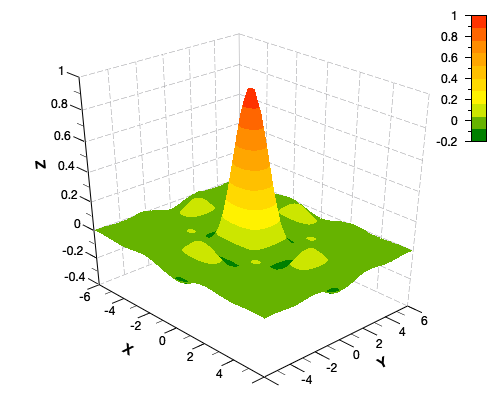A surface plot is like a wireframe plot, but each face of the wireframe is a filled polygon. QtiPlot uses OpenGL in order to achieve hardware-accelerated rendering of 3D surfaces as polygon meshes.

## 3D Vectors & TrajectoryQtiPlot can plot 3D vectors from two different data structures: XYZ XYZ and XYZ dXdYdZ.

The six XYZ XYZ data columns define the start and end points for the vectors, while XYZ dXdYdZ data columns provide the vector start points and the distances between the projections of the start and end points of the vectors on the three axes, X, Y and Z, respectively.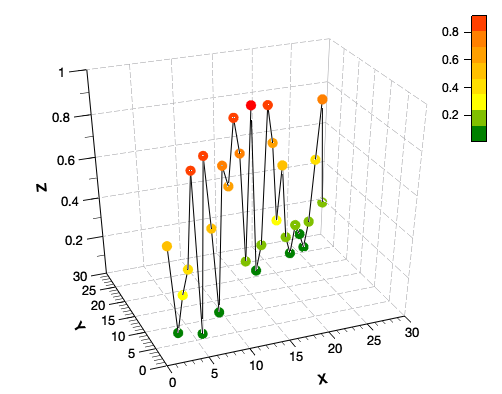The 3D trajectory and dots plot is one of the most basic plot types. It consists of a line that unites the 3D points created from (x, y, z) data triples.

Adding a colormap to the scatter points can aid the perception of the Z coordinate of the data being visualised.